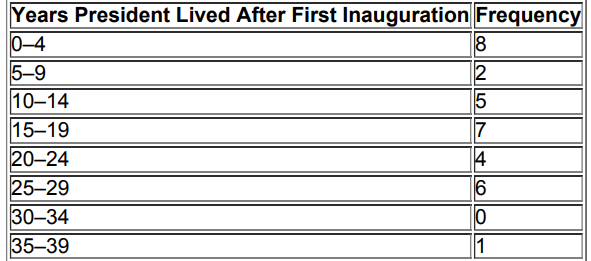×
Get Full Access to Elementary Statistics - 12 Edition - Chapter 3.3 - Problem 40bsc
Get Full Access to Elementary Statistics - 12 Edition - Chapter 3.3 - Problem 40bsc

×

# Solution: Finding Standard Deviation from a FrequencyISBN: 9780321836960 18

## Solution for problem 40BSC Chapter 3.3

Elementary Statistics | 12th Edition

• Textbook Solutions
• 2901 Step-by-step solutions solved by professors and subject experts
• Get 24/7 help from StudySoup virtual teaching assistantsElementary Statistics | 12th Edition

4 5 1 322 Reviews
15
3
Problem 40BSC

Finding Standard Deviation from a Frequency Distribution. In Exercises 37–40, find the standard deviation of sample data summarized in a frequency distribution table by using the formula below, where x represents the class midpoint, f represents the class frequency, and n represents the total number of sample values. Also, compare the computed standard deviations to these standard deviations obtained by using Formula 3­4 with the original list of data values: (Exercise 37) 11.1 years; (Exercise 38) 9.0 years; (Exercise 39) 13.4; (Exercise 40) 9.7 years.

s = n [ ∑ ( f ⋅ x 2 ) ] − [ ∑ ( f ⋅ x ) ] 2 n ( n − 1 ) Standard deviation for frequency distributionStep-by-Step Solution:
Step 1 of 2

Solution  40BSC

From the given information, the frequency distribution of years President lived after first inauguration is

 Years President Lived after first Inauguration Frequency 0 – 4 8 5 – 9 2 10 – 14 5 15 – 19 7 20 – 24 4 25 – 29 6 30 – 34 0 35 – 39 1

Step 2 of 2

##### ISBN: 9780321836960

Unlock Textbook Solution